### Home > CAAC > Chapter 13 > Lesson 13.OF1-S > Problem7-132

7-132.
1. Use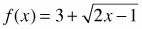to complete parts (a) through (e) below. Homework Help ✎

1. What are the domain and range of f(x)?

2. What is the inverse of f(x)? Call it g(x).

3. What are the domain and range of g(x)?

4. Find an expression for f(g(x)).

5. Find an expression for g(f(x)). What do you notice? Why does this happen?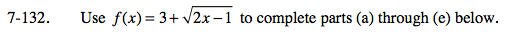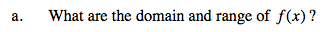Which values of x will cause the square root expression to be undefined?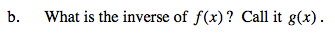If f(x) = y, then switch the x and the y in f(x). Solve for y.

$\textit{g}(\textit{x})=\frac{(\textit{x}-3)^{2}+1}{2}$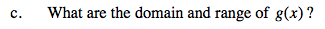Switch the domain and range of f(x).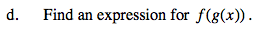Substitute the equation g(x) that you found in part (b) for x in f(x).

$f(g(x))=3+\sqrt{2\left(\frac{(x-3)^{2}+1}{2}\right)-1}$

Simplify.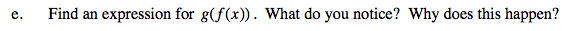See part (d). What relationship do f(x) and g(x) have with each other? (What is the relationship between two inverses?)